## Respiratory Sounds Used with Spectrum Analysis

Weiss EB, Carlson C.J. Recording of breath sounds. [PDF] Am Rev Respir Dis. 1972; 105:835-839.

Here are some medical-related sounds that we looked at with the spectrum analyzer. The first seven are from the 'Introduction to Breath Sounds' cassette tape by David W. Cugell, M.D. with Earle B. Weiss M.D. Also included is a graphic of each sound spectra similiar to that as seen by the Spectrum Analyzer, and it's corresponding waveform.

All sound files are 10.000 seconds long digitized at a sampling rate of 11025 Hz in mono.

#### Normal Inspiration Sound [WAV]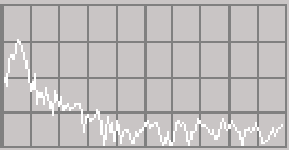Spectra of the normal inspiration sound file at exactly 3.324 seconds similiar to that seen by the HP Spectrum Analyzer. From left to right, each bar represents a span of 689 Hz up to 5512 Hz (half the sampling rate.) That is, the first bar is from 0 to 689 Hz, the second from 690 to 1379 Hz and so on with the last bar representing 4824 to 5512 Hz.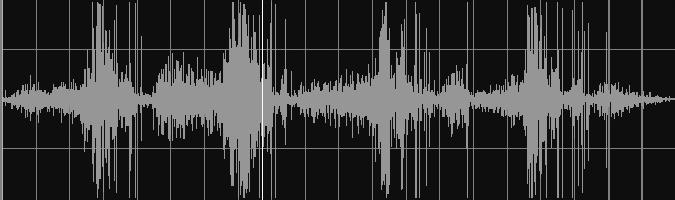Sound waveform of the Normal Inspiration sound. The horizontal axis is time. The file is exactly 10.000 seconds long.

#### Asthma Attack Sound [WAV]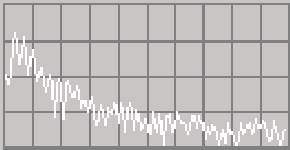Spectra of the Asthma Attack sound file at exactly 6.333 seconds similiar to that seen by the HP Spectrum Analyzer. From left to right, each bar represents a span of 689 Hz up to 5512 Hz (half the sampling rate.) That is, the first bar is from 0 to 689 Hz, the second from 690 to 1379 Hz and so on with the last bar representing 4824 to 5512 Hz.Sound waveform of the Asthma Attack sound. The horizontal axis is time. The file is exactly 10.000 seconds long.

#### Bronchial Breathing Sound [WAV]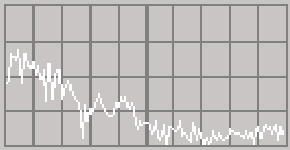Spectra of the Bronchial Breathing sound file at exactly 3.253 seconds similiar to that seen by the HP Spectrum Analyzer. From left to right, each bar represents a span of 689 Hz up to 5512 Hz (half the sampling rate.) That is, the first bar is from 0 to 689 Hz, the second from 690 to 1379 Hz and so on with the last bar representing 4824 to 5512 Hz.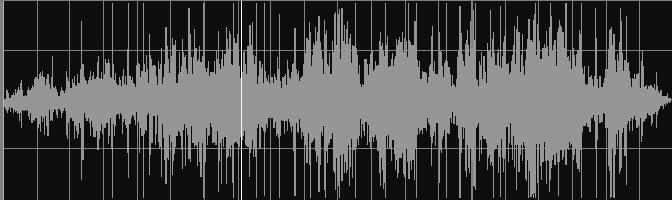Sound waveform of the Bronchial Breathing sound. The horizontal axis is time. The file is exactly 10.000 seconds long.

#### Plural Friction Sound [WAV]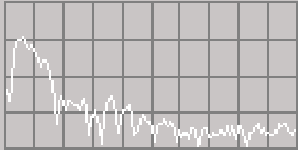Spectra of the Plural Friction sound file at exactly 2.574 seconds similiar to that seen by the HP Spectrum Analyzer. From left to right, each bar represents a span of 689 Hz up to 5512 Hz (half the sampling rate.) That is, the first bar is from 0 to 689 Hz, the second from 690 to 1379 Hz and so on with the last bar representing 4824 to 5512 Hz.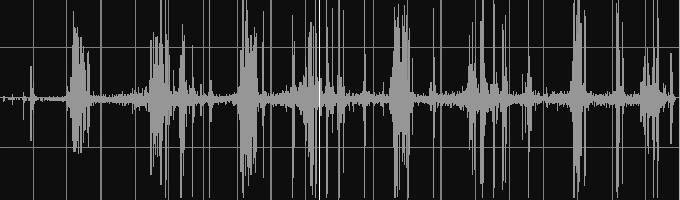Sound waveform of the Plural Friction sound. The horizontal axis is time. The file is exactly 10.000 seconds long.

#### Medium Rales Sound [WAV]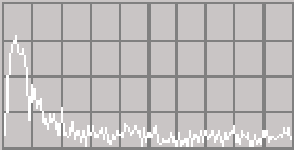Spectra of the Medium Rowls sound file at exactly 7.059 seconds similiar to that seen by the HP Spectrum Analyzer. From left to right, each bar represents a span of 689 Hz up to 5512 Hz (half the sampling rate.) That is, the first bar is from 0 to 689 Hz, the second from 690 to 1379 Hz and so on with the last bar representing 4824 to 5512 Hz.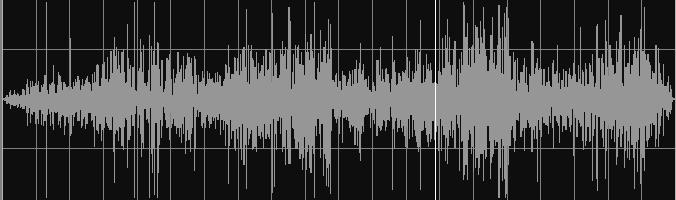Sound waveform of the Medium Rowls sound. The horizontal axis is time. The file is exactly 10.000 seconds long.

#### More Bronchial Breathing Sound [WAV]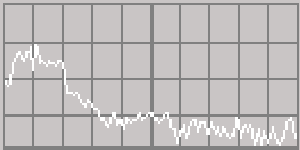Spectra of the Bronchial Breathing sound file at exactly 3.674 seconds similiar to that seen by the HP Spectrum Analyzer. From left to right, each bar represents a span of 689 Hz up to 5512 Hz (half the sampling rate.) That is, the first bar is from 0 to 689 Hz, the second from 690 to 1379 Hz and so on with the last bar representing 4824 to 5512 Hz.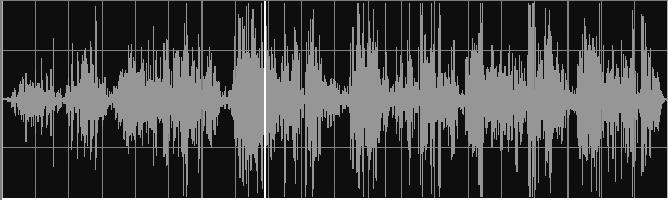Sound waveform of the More Bronchial Breathing sound. The horizontal axis is time. The file is exactly 10.000 seconds long.

#### Fluid In Lungs Breathing Sound [WAV]Spectra of the Normal inspiration sound file at exactly 5.769 seconds similiar to that seen by the HP Spectrum Analyzer. From left to right, each bar represents a span of 689 Hz up to 5512 Hz (half the sampling rate.) That is, the first bar is from 0 to 689 Hz, the second from 690 to 1379 Hz and so on with the last bar representing 4824 to 5512 Hz.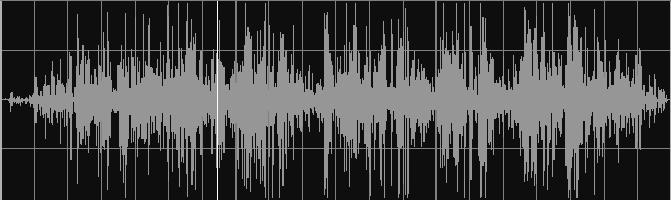Sound waveform of the Fluid in Lungs sound. The horizontal axis is time. The file is exactly 10.000 seconds long.